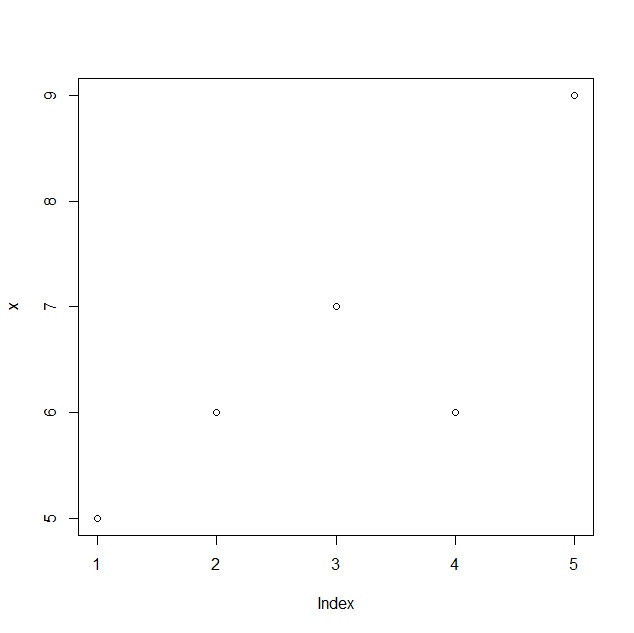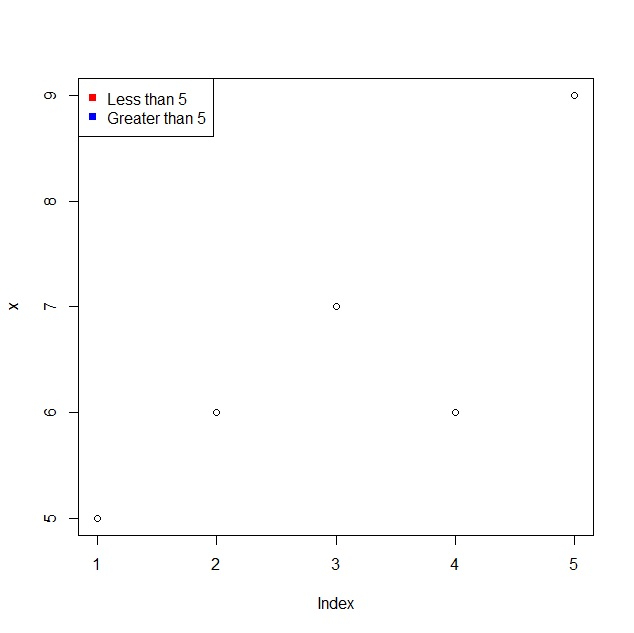# How to display legend in base R with different colors?

R ProgrammingServer Side ProgrammingProgramming

To display legend in base R with different colors, we can follow the below steps −

• First of all, create a plot using plot function.

• Then, use legend function legend argument and col argument to display legend with different colors.

## Example

#### Create the plot

Let’s create a plot of a vector x using plot function −

x<-rpois(5,5)
plot(x)

## OutputDisplay legend with different colors

Using legend function legend argument and col argument to display legend with red and blue colors −

x<-rpois(5,5)
legend("topleft",legend=c("Less than 5","Greater than 5"),pch=15,col=c("red","blue"))

## Output